# Differential and Integral Calculus - 1 Notes | Study Business Mathematics and Logical Reasoning & Statistics - CA Foundation

## CA Foundation: Differential and Integral Calculus - 1 Notes | Study Business Mathematics and Logical Reasoning & Statistics - CA Foundation

The document Differential and Integral Calculus - 1 Notes | Study Business Mathematics and Logical Reasoning & Statistics - CA Foundation is a part of the CA Foundation Course Business Mathematics and Logical Reasoning & Statistics.
All you need of CA Foundation at this link: CA Foundation

1. Introduction and highlights

Diferential calculus you learned in the past term was about dierentiation. You may feel embarrassed to nd out that you have already forgotten a number of things that you learned differential calculus. However, if you still remember that differential calculus was about the rate of change, the slope of a graph, and the tangent of a curve, you are probably OK.

• The essence of differentiation is nding the ratio between the difference in the value of f (x) and the increment in x.

Remember, the derivative or the slope of a function is given by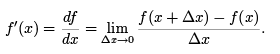......(1)

Integral calculus that we are beginning to learn now is called integral calculus. It will be mostly about adding an incremental process to arrive at a \total". It will cover three major aspects of integral calculus:

1. The meaning of integration.

We'll learn that integration and differentiation are inverse operations of each other.
They are simply two sides of the same coin (Fundamental Theorem of Caclulus).

2. The techniques for calculating integrals.

3. The applications.

2. Sigma Sum

In essence, integration is an advanced form of addition. We all started learning how to add two numbers since as young as we could remember. You might say ? Are you kidding? Are you telling me that I have to start my university life by learning addition?".

The answer is positive. You will nd out that doing addition is often much harder than calculating an integral. Some may even nd sigma sum is the most dicult thing to learn in integral calculus. Although this diffculty is by-passed by using the Fundamental Theorem of Caclulus, you should NEVER forget that you are actual ly doing a sigma sum when you are calculating an integral. This is one secret for correctly formulating the integral in many applied problems with ease!

Now, I use a couple of examples to show that your skills in doing addition still need improvement.

Example 1a: Find the total number of logs in a triangular pile of four layers (see figure).

Solution 1a: Let the total number be S4, where S' stands for Sum' and the subscript reminds us that we are calculating the sum for a pile of 4 layers.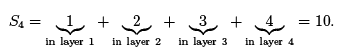A piece of cake!

Example 1b: Now, find the total number of logs in a triangular pile of 50 layers, i.e. nd S50 ! (Give me the answer in a few seconds without using a calculator).

Solution 1b: Let's start by formulating the problem correctly.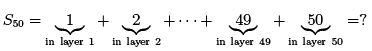where `..... ' had to be used to represent the numbers between 3 and 48 inclusive. This is because there isn't enough space for writing all of them down. Even if there is enough space, it is tedious and unnecessary to write all of them down since the regularity of this sequence makes it very clear what are the numbers that are not written down.

Still a piece of cake? Not really if you had not learned Gauss's formula. We'll have to leave it unanswered at the moment.

Example 2: Finally, nd the total number of logs in a triangular pile of k layers, i.e. nd Sk (k is any positive integer, e.g. k = 8; 888; 888 is one possible choice)!

Solution: This is equivalent to calculating the sum of the fifirst k positive integers.

Sk = 1 + 2 + ...... + (k 1) + k.

The only thing we can say now is that the answer must be a function of k which is the total number of integers we need to add. Again, we have to leave it unanswered at the moment.

2.2 Regular vs irregular sequences

A sequence is a list of numbers written in a de nite order. A sequence is regular if each term of the sequence is uniquely determined, following a well-de ned rule, by its position/order in the sequence (often denoted by an integer i). Very often, each term can be generated by an explicit formula that is expressed as a function of the position i, e.g. f (i). We can call this formula the sequence generator or the general term.

For example, the ith term in the sequence of integers is identical to its location in the sequence, thus its sequence generator is f (i) = i. Thus, the 9th term is 9 while the 109th term is equal to 109.

Example 3: The sum of the first ten odd numbers is

O10 = 1 + 3 + 5 + .......+ 19.

Find the sequence generator.

Solution 3: Note that the ith odd number is equal to the ith even number minus 1. The ith even number is simply 2i. Thus, the ith odd number is 2i - 1, namely f (i) = 2i - 1. To verify, 5 is the 3rd odd number, i.e. i = 3. Thus, 2i - 1 = 2 x 3 - 1 = 5 which is exactly the number we expect.

Knowing the sequence generator, we can write down the sum of the fifirst k odd numbers for any positive integer k.

Ok = 1 + 3 + 5 + .......+ (2k - 1).

Example 4: Find the sequence generator of the following sum of 100 products of subsequent pairs of integers.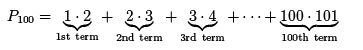Solution 4: Since the ith term is equal to the number i multiplied by the subsequent integer which is equal to i + 1. Thus, f (i) = i(i + 1).

Knowing the sequence generator, we can write down the sum of k such terms for any positive integer k.

Pk = 1.2 + 2.3 + .......+ k(k + 1).

Example 4: The sequence of the fifirst 8 digits of the irrational number π = 3:1415926 ...... is

π8 = 3 + 1 + 4 + 1 + 5 + 9 + 2 + 6.

We cannot nd the sequence generator since the sequence is irregular, we cannot express the sum of the first k digits of π(for arbitrary k)

2.3 The sigma notation

In order to short-hand the mathematical exression of the sum of a regular sequence, a convenient notation is introduced.

Definition (∑ sum): The sum of the first k terms of a sequence generated by the sequence generator f (i) can be denoted by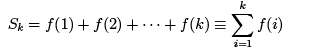where the symbol (the Greek equivalent of S reads \sigma") means \take the sum of ", the general expression for the terms to be added or the sequence generator f (i) is called the summand, i is called the summation index, 1 and k are, respectively, the starting and the ending indices of the sum.
Thus,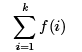means calculate the sum of all the terms generated by the sequence generator f (i) for all integers starting from i = 1 and ending at i = k.

Note that the value of the sum is independent of the summation index i, hence i is called a "dummy" variable serving for the sole purpose of running the summation from the starting index to the ending index. Therefore, the sum only depends on the summand and both the starting and the ending indices.

Example 5: Express the sum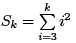in an expanded form.

Solutions: The sequence generator is f (i) = i2 . Note that the starting index is not 1 but 3!. Thus, the 1st term is f (3) = 32. The subsequent terms can be determined accordngly. Thus,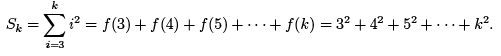An easy check for a mistake that often occurs. If you still nd the \dummy" variable i in an expanded form or in the nal evaluation of the sum, your answer must be WRONG.

2.4 Gauss's formula and other formulas for simple sums

Let us return to Examples 1 and 2 about the total number of logs in a triangular pile. Let's start with a pile of 4 layers. Imaging that you could (in a \thought-experiement") put an identical pile with up side down adjacent to the original pile, you obtain a pile that contains twice the number of logs that you want to calculate (see figure).

The advantage of doing this is that, in this double-sized pile, each layer contains an equal number of logs. This number is equal to number on the 1st (top) layer plus the number on the 4th (bottom) layer. In the mean time, the height of the pile remains unchanged (4). Thus, the number in this double-sized pile is 4 x (4 + 1) = 20. The sum S4 is just half of this number which is 10.

Let's apply this idea to nding the formula in the case of k layers. Note that

(Original) S= 1 + 2 + ....... + k - 1 + k ....... (2)

(Inversed) Sk = k + k - 1 + ...... + 2 + 1..........(3)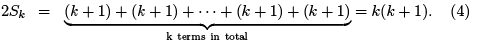Dividing both sides by 2, we obtain Gauss's formula for the sum of the first k positive integers.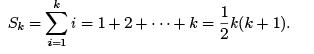.....(5)

This actaully answered the problem in Example 2. The answer to Example 1b is even simpler.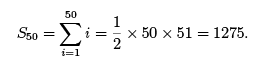The following are two important simple sums that we shall use later. One is the sum of the fifirst k integers squared.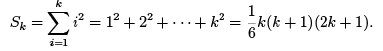......(6)

The other is the sum of the fifirst k integers cubed.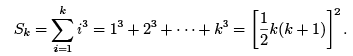.......(7)

We shall not illustrate how to derive these formulas. You can nd it in numerous calculus text books.

To prove that these formulas work for arbitrarily large integers k, we can use a method called mathematical induction. To save time, we'll just outline the basic ideas here.

The only way we can prove things concerning arbitrarily large numbers is to guarantee that this formula must be correct for k = N + 1 if it is correct for k = N . This is like trying to arrange a string/sequence of standing dominos. To guarantee that all the dominos in the string/sequence fall one after the other, we need to guarantee that the falling of each domino will necessarily cause the falling of the subsequent one. This is the essence of proving that if the formula is right for k = N , it must be right for k = N + 1. The last thing you need to do is to knock down the first one and keep your fingers crossed. Knocking down the first domino is equivalent to proving that the formula is correct for k = 1 which is very easy to check in all cases (see Keshet's notes for a prove of the sum of the first k integers squared).

2.5 Important rules of sigma sums

Rule 1: Summation involving constant summand.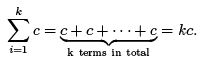Note that: the total # of terms = ending index - starting index +1.

Rule 2: Constant multiplication: multiplying a sum by a constant is equal to multiplying each term of the sum by the same constant.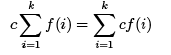Rule 3: Adding two sums with identical starting and ending indices is equal to the sum of sums of the corresponding terms.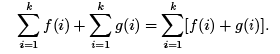Rule 4: Break one sum into more than one pieces.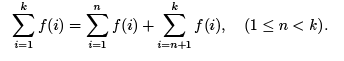Example 7: Let's go back to solve the sum of the rst k odd numbers.

Solution 7: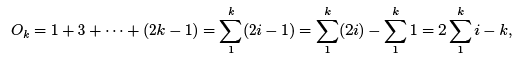where Rules 1, 2, and 3 are used in the last two steps. Using the known formulas, we obtain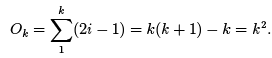Example 8: Let us now go back to solve Example 4. It is the sum of the first k products of pairs of subsequent integers.

Solution 8:

Pk = 1 . 2 + 2 . 3 + ...... + k .(k + 1)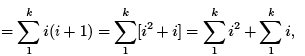where Rule 3 was used in the last step. Applying the formulas, we learned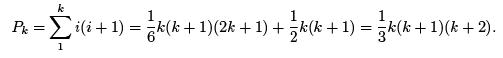This is another simple sum that we can easily remember.

Example 9: Calculate the sum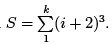Solution 9: This problem can be solved in two different ways. The rst is to expand the summand f (i) = (i + 2)3 which yield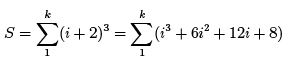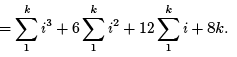We can solve the resulting three sums separately using the known formulas.

But there is a better way to solve this. This involves substituting the summation index. We nd it easier to see how substitution works by expanding the sum.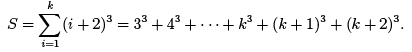We see that this is simply a sum of integers cubed. But the sum does not start at 1and end at k3 like in the formula for the sum of the first k integers cubed.

Thus we re-write the sum with a sigma notation with an new index called l which starts at l = 3 and ends at l = k + 2 (there is no need to change the symbol for the index, you can keep calling it i if you do not feel any confusion). Thus,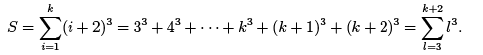We just finished doing a substitution of the summation index. It is equivalent to replacing i by l = i + 2. This relation also implies that i = 1 , l = 3 and i = k ⇒ l = k + 2. This is actually how you can determine the starting and the ending values of the new index

Now we can solve this sum using Rule 4 and the known formula.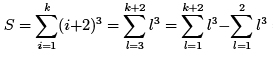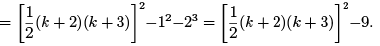2.6 Applications of sigma sum

The area under a curve

We know that the area of a rectangle with length l and width w is Arect = w.l.

Starting from this formula we can calculate the area of a triangle and a trapezoid. This is because a triangle and a trapezoid can be transformed into a rectangle (see Figure). Thus, for a triangle of height h and base length b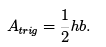Similarly, for a trapezoid with base length b, top length t, and height h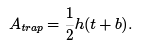Following a very similar idea, the sum of a trapezoid-shaped pile of logs with t logs on top layer, b logs on the bottom layer, and a height of h = b - t + 1 layers (see figure) is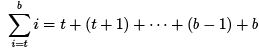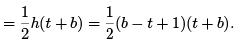.....(8)

Now returning to the problem of calculating the area. Another important formula is for the area of a circle of radius r.

Acirc = πr2

Now, once we learned sigma and/or integration, we can calculate the area under the curve of any function that is integrable.

Example 10: Calculate the area under the curve y = x2 between 0 and 2 (see figure).

Solution 10: Remember always try to reduce a problem that you do not know how to solve into a problem that you know how to solve.

Let the area be A. Let's divide A into 3 rectangles of equal width w = 2/3. Thus,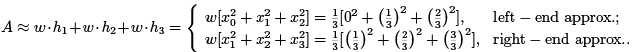By using these rectangles, we introduced large errors in our estimates using the heights based on both the left and the right endpoints of the subintervals. To increase accurary, we need to increase the number of rectangles by making each one thinner. Let us now divide A into n rectangles of equal width w = 2\n. Thus, using the height based on the right endpoint of each subinterval, we obtain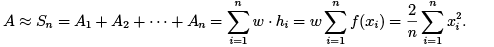It is very important to keep a clear account of the height of each rectangle. In this case, hi = f (xi) = x2i . Thus, the key is nding the x-coordinate of the right-end of each rectangle. For rectangles of of equal width, x= x+ iw = x+ iΔx/n, where Δx is the length of the interval x0 is the left endpoint of the interval. x0 = 0 and Δx= 2 for this example. Therefore, xi = i(2/n). Substitute into the above equation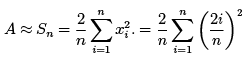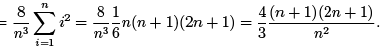Note that if we divide the area into in nitely many retangles with a width that is in nitely small, the approximate becomes accurate.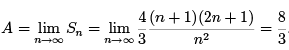The volume of a solid revolution of a curve

We know the volume of a rectangular block of height h, width w, and length l is

Vrect = w.l .h

The volume of a cylinder of thichness h and radius r

Vcyl = Across . h = πr2h.

Example 11: Calculate the volume of the bowl-shaped solid obtained by rotating the curve y = x2 on [0; 2] about the y-axis.

The document Differential and Integral Calculus - 1 Notes | Study Business Mathematics and Logical Reasoning & Statistics - CA Foundation is a part of the CA Foundation Course Business Mathematics and Logical Reasoning & Statistics.
All you need of CA Foundation at this link: CA FoundationUse Code STAYHOME200 and get INR 200 additional OFF

## Business Mathematics and Logical Reasoning & Statistics

81 videos|101 docs|87 tests

Track your progress, build streaks, highlight & save important lessons and more!

,

,

,

,

,

,

,

,

,

,

,

,

,

,

,

,

,

,

,

,

,

;